International Journal of Systems Science and Applied Mathematics
Volume 1, Issue 4, November 2016, Pages: 50-57

Comparative Analysis of Numerical Solution to a Linear System of Algebraic Equations

Aliyu Bhar Kisabo1, *, Aliyu Adebimpe Funmilayo1, Major Kwentoh Augustine Okey2

1Center for Space Transport & Propulsion, Instrumentation & Control Department Epe, Nigeria

2Hedquarters Nigerian Army Corps of Electrical and Mechanical Engineers Bonny Cantonment VI, Nigeria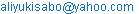(A. B. Kisabo)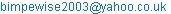(A. A. Funmilayo)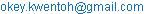(K. A. Okey)

*Corresponding author

Aliyu Bhar Kisabo, Aliyu Adebimpe Funmilayo, Major Kwentoh Augustine Okey. Comparative Analysis of Numerical Solution to a Linear System of Algebraic Equations. International Journal of Systems Science and Applied Mathematics. Vol. 1, No. 4, 2016, pp. 50-57. doi: 10.11648/j.ijssam.20160104.13

Received: September 28, 2016; Accepted: October 9, 2016; Published: October 31, 2016

Abstract: In engineering and science, linear systems of algebraic equations occur often as exact or approximate formulations of various problems. These types of equations are well represented in matrix form. A major challenge for researchers is the choice of algorithm to use for an appropriate solution. In this study, we choose to experiment with three algorithms for the solution to a system of linear algebraic equation. After subjecting the matrix form of the system of linear algebraic equations to the rank test, Gaussian Elimination method, Inverse Matrix Method and Row-Reduced Echelon were used to evaluate twenty-four (24) sets of solutions. Numerical methods are plagued by truncation and round-off errors thus, we choose to compute and compare result here by invoking the MATLAB command format long (15 decimal place) with format short (5 decimal place). After evaluating the required solutions, we substituted all computed results back into the system of linear algebraic equations to check if they are satisfied. Comparison of results was done on the basis of algorithm used and between the results obtained using either format long or format short values. Despite the presence of errors due to truncation and round-off, format short computed solutions gave acceptable result in some cases. Results obtained in this study proved the efficacy of the proposed technique.

Keywords: Linear System of Algebraic Equations, Numerical Methods, MATLAB®

Contents

1. Introduction

The most important treatise in the history of Chinese mathematics is the Chiu Chang Chiu Chang Suan Shu in Chinese characters or "The Nine Chapters of the Mathematical Art." This treatise, which is a collection of 246 problems and their solutions, was assembled in its final form by Liu Hui in A.D. 263. Its contents, however, go back to at least the beginning of the Han dynasty in the second century B.C. The eighth of its nine chapters, entitled "The Way of Calculating by Arrays," contains 18 word problems that lead to linear systems in three to six unknowns. The general solution procedure described is almost identical to the Gaussian Elimination technique developed in Europe in the nineteenth century by Carl Friedrich Gauss .

Harvard Professor Wassily Leontief was carefully feeding the last of his punched cards into the University’s Mark II computer. The cards contained information about the U.S. economy and represented a summary of more than 250,000 pieces of information produced by the U.S. Bureau of Labor Statistics after two years of intensive work. Leontief had divided the U.S. economy into 500 "sectors," such as the coal industry, the automotive industry, communications, and so on.

For each sector, he had written a linear equation that described how the sector distributed its output to the other sectors of the economy. One of the largest computers of its day, the Mark II, could not handle the resulting system of 500 equations in 500 unknowns, Leontief had distilled the problem into a system of 42 equations in 42 unknowns.

Leontief’s Nobel Prize awarded in 1973 opened the door to a new era in numerical mathematical modeling. His efforts at Harvard in 1949 marked one of the first significant uses of computers to analyze what was then a largescale mathematical model. Since that time, researchers in many other fields have employed computers to analyze mathematical models. Because of the massive amounts of data involved, the models are usually linear; that is, they are described by systems of linear equations.

Today, linear algebra has more potential value for researchers in many scientific and business fields than any other mathematics subject. Some areas of its are :

Oil exploration. When a ship searches for offshore oil deposits, its computers solve thousands of separate systems of linear equations every day. The seismic data for the equations are obtained from underwater shock waves created by explosions from air guns. The waves bounce off subsurface rocks and are measured by geophones attached to

mile-long cables behind the ship

Linear programming. Many important management decisions today are made on the basis of linear programming models that use hundreds of variables. The airline industry, for instance, employs linear programs that schedule flight crews, monitor the locations of aircraft, or plan the varied schedules of support services such as maintenance and terminal operations.

Electrical networks. Engineers use simulation software to design electrical circuits and microchips involving millions of transistors. Such software relies on linear algebra techniques and systems of linear equations.

The importance of linear algebra for applications has risen in direct proportion to the increase in computing power, with each new generation of hardware and software triggering a demand for even greater capabilities. Computer science is thus intricately linked with linear algebra through the explosive growth of parallel processing and large-scale computations. Scientists and engineers now work on problems far more complex than even dreamed possible a few decades ago.

The concept of linear algebra in matrix form have widespread usage in many areas of science and engineering especially over the last three decades. With the aid of computers, matrices have been employed effectively in many applications ranging from signal processing, controls, finite elements analysis, communications, computer vision, electromagnetics, social and health sciences etc. This fueled the development of several matrices-based analytical packages such as MATLAB® to help engineers and scientists simulate or solve large systems numerically using fundamental linear algebra concept.

2. Numerical Solution

One of the most reliable aspects of numerical analysis programs for the electronic digital computer is that they always produce numbers. As a result of the considerable reliability of the machines, it is common to regard the results of their calculations with a certain air of infallibility. However, the results can be no better than the method of analysis and implementation program utilized by the computer. This is the origin of the aphorism "garbage in garbage out". Because of the large number of calculations carried out by these machines, small errors at any given stage can rapidly propagate into large ones that destroy the validity of the result.

Computers store real numbers in floating-point form. In general, the floating-point form of a number is ±M x10k, where k is an integer and the mantissa M is a (decimal) real number that satisfies 0.1 ≤ M < 1. The maximum number of decimal places that can be stored in the mantissa depends on the computer. If the maximum number of decimal places that can be stored is d, we say that there are d significant digits. Any digits that are not stored are either omitted (in which case we say that the number has been truncated) or used to round the number to d significant digits.

Digital computers utilize a certain number of digits in their calculations and this base number of digits is known as the precision of the machine. Often it is possible to double or triple the number of digits and hence the phrase "double" or "triple" precision is commonly used to describe a calculation carried out using this expanded number of digits. It is common practice to use more digits than are justified by the problem simply to be sure that one has "got it right". Consider the common 6-digit machine. It will be unable to distinguish between 1 million dollars and 1 million and nine dollars. Subtraction of those two numbers would yield zero. This would be significant to any accountant at a bank. Repeated operations of this sort can lead to a completely meaningless result in the first digit.

Whenever truncation or rounding occurs, a round-off error is introduced, which can have a dramatic effect on the calculations . Therefore, we must be careful about the propagation of round-off error into the final computational result. We must keep in mind that both round-off and truncation errors will be present at some level in any calculation and be wary lest they destroy the accuracy of the solution. The acceptable level of accuracy is determined by the analyst and he must be careful not to aim too high and carry out grossly inefficient calculations, or too low and obtain meaningless results .

In this study, first we argue that it is only by experimenting with different algorithms and comparing results that a satisfactory solution can be reached. Second, we make lucid the effect of round-off error in such computations. These we shall explore by numerically solving a system of linear algebraic equation describing current flow in a simple circuit.

3. Linear System of Algebraic Equation

Linear algebra has evolved as a branch of mathematics with a wide range of applications in natural sciences, engineering, computer sciences, management and social sciences, and more. Many problems in the sciences lead to solving more than one linear equation. The general situation can be described by a linear system. A system of linear equations or simply a linear system is any finite collection of linear equations. A linear system of m equations in n variables

has the form described in (1).

A linear system can have infinitely many solutions (dependent system), exactly one solution (independent system) or no solutions at all. When a linear system has a solution we say that the system is consistent. Otherwise, the system is said to be inconsistent.

Suppose F is a field. We consider the problem of finding n scalars (elements of F) x1,…,xn which satisfy the conditions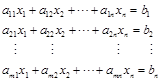(1)

where b1,…,bn and aij, such that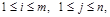are given elements of F. then we call (1) a system of m linear equations in n unknowns. Any n-tuple (x1,…,xn) of elements of F which satisfies each of (1) is called a solution of the system. If b1 = b2 =…= bm = 0, we say that the system is homogeneous, or that each of the equation is homogeneous.

In concise form, (1) can be written as(2)

where aij, denotes the coefficient of the jth unknown xj in the ith equation, and the numbers aij, and bi, (hence xj) all are real. Often the number r of equations is equal to the number n of unknowns, but exceptions are common in optimization and modeling. In matric form, (1) can be represented as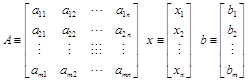(3)

Note, A denotes the matrix with coefficients aij,, x the unknown column vector and b the right hand column vector. Another way of representing an array is to enclose the expression for its elements in curly brackets: A = { aij }, x = {xj}, b = {bi}. Defining the matrix-vector product(4)

In accordance with (2), one can summaries (1) concisely as ,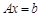(5)

Methods for solving (1) can then be described in terms of operations on the arrays A and b, (1) has solution if and only if b is a linear combination of nonzero column vectors of A; then we say that b is in the column space of A. In such cases, if the rank r, of A equal’s n there is a unique solution, whereas if r < n there is an infinity of solutions characterized by (n - r) free parameters.

4. Methods of Solution

Sets of linear algebraic equations can be expressed as a single equation, using matrix notation as given in (3). This standard and compact form is useful for expressing solutions and for developing software applications with an arbitrary number of variables. Matrix notation enables us to represent multiple equations as a single matrix equation.

There exist several algorithms for the solution of linear algebraic system of equations. Only three will be discussed here based on the fact that they will be used to solve the selected problem.

4.1. Matrix Inversion

The situation is simplest if the matrix A is square (r = n); then a unique solution vector x exists if and only if the rank of A equals its order n. Such a matrix A has a unique inverse, A-l, defined by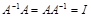(6)

and is said to be nonsingular or regular. Here I is the unit matrix expressed in (7) of the same order as A.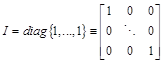(7)

A necessary and sufficient condition for matrix A to be nonsingular is that the determinant of A be nonzero. Under this condition, A− 1 is computed using the following equation :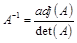(8)

where adj(A) stands for the adjoint matrix of A which is defined to be the n x n matrix of cofactors given by: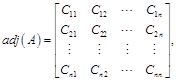(9)

where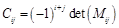(10)

and Mij is the minor corresponding to aij and is defined as the (n− 1) x (n− 1) matrix obtained by eliminating the ith row and jth column of A.

The inverse of a matrix A is defined only if A is square and nonsingular. A matrix is singular if its determinant |A| is zero. In general, the determinant of an n x n matrix A is defined as: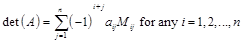(11)

where Mij is the minor corresponding to aij and is the determinant of the matrix obtained by deleting the row and column containing aij. Let Aij = (− 1)i+ jMij, where Aij is defined as the cofactor of aij. Then: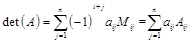(12)

When the inverse of a matrix is computed, then the solution to the linear system of algebraic equation is evaluated as,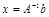(13)

Thus in MATLAB, the syntax inv(A)* b will produce the solution required as described by (13). This syntax implements an inverse matrix algorithm for the system of linear algebraic equations. In MATLAB a result will be obtained provided the rank r, of A equals n.

4.2. Gaussian Elimination

Consider a set of linear equations as given in (5), where x and b represent n x 1 vectors, and A is an n x n matrix. Another approach to solve the system of linear equations Ax = b is by utilizing the Gaussian elimination technique. In this method, we combine matrix A and column vector b into an augmented n x (n + 1) matrix as follows: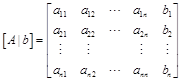(14)

A sequence of elementary row operations is applied to this augmented matrix to transform the matrix into an upper triangular matrix of the form: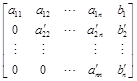(15)

Now, we can solve the last equation for the unknown (xn) and substitute it back into the previous equation to obtain a solution for xn − 1. This process is continued using back-substitution until all of the unknowns have been found. The general solution is given by: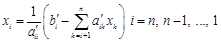(16)

For computation of a particular solution vector, this method requires 1/3 (n3- n) + n2 operations of multiplication or division .

MATLAB provides the left division method for solving (5) based on Gauss Elimination. To use the left division method to solve for x, you type x = A\b. If |A| = 0 or if the number of equations does not equal the number of unknowns, then you need to use the other methods to be presented later.

4.3. The Row-Reduced Echelon Method

A matrix is said to be in row echelon form if it satisfies all of the following:

The first nonzero entry in each nonzero row is 1.

If the kth row does not consist entirely of zeros, then the number of leading zero entries in the (k + 1)th row should be greater than the number of leading zero entries in the kth row.

If there are any rows whose entries are all zero, they should be below the rows with nonzero entries.

One can also describe an m x n row–reduced echelon matrix R as follows . Either every entry in R is 0, or there exists a positive integer r, 1 ≤ rm, and r positive integers k1,…, kr with 1 ≤ kin and

Rij = 0 for i > r, and Rij = 0 for j < ki

Riki = δij, 1 I ≤ r, 1 ≤ j ≤ r

k1 < … < kr

In MATLAB, the rref function provides a procedure for reducing an equation set to this form, which is called the row-reduced echelon form. Its syntax is rref([A b]). Its output is the augmented matrix [C d] that corresponds to the equation set Cx = d. This set is in row-reduced echelon form

5. Solving a Typical Problem

A typical problem of linear system of algebraic equation is gotten from  and is as follows:

Equation (19) describes the circuit shown in Fig. 1.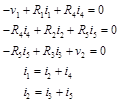(17)Fig. 1. Electrical circuit.

(a)    The given values of the resistances and the voltage v1 are R1 =5, R2 = 100, R3=200, R4=150, R5=250 k, and v1 =100 V. (Note that 1 kΩ =1000 .) Suppose that each resistance is rated to carry a current of no more than 1 mA (=0.001A). Determine the allowable range of positive values for the voltage v2.

(b)    Suppose we want to investigate how the resistance R3 limits the allowable range for v2. Obtain a plot of the allowable limit on v2 as a function of R3 for 150 ≤ R3 ≤ 250 kΩ.

To obtain the solution to (17), it has to be re-written to account for the all other relating variables of current. Also, the numerical values of resistance must be captured and terms re-arranged, this gives;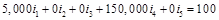(18)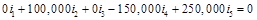(19)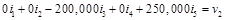(20)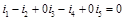(21)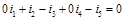(22)

In matrix form [10-12], (18) -(22) is presented as given in (23). Note that here we assume v2 = 1V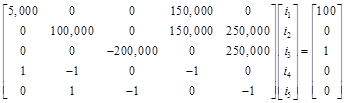(23)

From (23), the following matrices emerge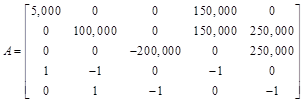(24)(25)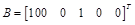(26)

The matrix A in (24) is square and det(A) ≠ 0. This implies that the Matrix Inversion method can be applied to it to obtain the needed solution. This method executed by the syntax inv(A)* b will warn us if a unique solution does not exist, but it does not tell us whether there is no solution or an infinite number of solutions. For this reason, it is prudent to subject the A and b matrixes in (24) and (26) to the rank of a matrix test.

This test suggests that if A is square and of dimension n x n, then rank ([A b]) = rank(A), and a unique solution exists for any b if rank(A) = n. For the problem under consideration A is a 5 x 5 matrix and rank(A) = rank ([A b]) = 5, hence, a unique solution exists. Gaussian Elimination method and Matrix Inverse Method can be employed for the solution required. But the question comes with a condition that says v2 can take a range of positive values. Any range of positive values can have infinite numbers. One will therefore be tempted to consider the problem as one with infinitely many solutions that is limited by a current of 0.001A. The implication of an infinitely many solutions for a set of linear algebraic equation will allude to the use of the Row-Reduced Echelon method, despite the fact that r = n.

This dilemma as to the choice of the most appropriate numerical algorithm for one to use can be surmounted by employing all three algorithms. Then by substituting the obtained solutions into the set of linear algebraic equations to check for its satisfaction.

First we choose to obtain solution to (24) and (26) in 5 decimal place and then in 15 decimal places by invoking the command format short and format long respectively in MATLAB.

All computations were done using R2016a version of MATLAB, on a 64-bit Windows 7 Laptop with Intel(R) Core(TM) i5-2430M @ 2.40GHz and RAM 6.00GB.

Applying Matrix Inverse and Gaussian Elimination Methods using the syntaxes x1=A\b and x2= inv(A)*b respectively gave the current values in (27) and (28).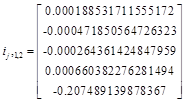(27)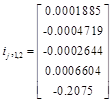(28)

where j =1…5 and j1,2 represents the currents obtained by methods 1, Matrix Inversion and method 2, Gaussian Elimination.

The Row-Reduced Echelon Method was used to solve the set of linear algebraic equations as represented in (24) and (26), using the MATLAB syntax x3 = rref([A, b]). An augmented matrix [C d] was obtained as;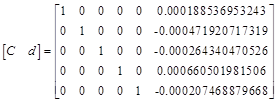(29)

The matrix in (30) corresponds to the matrix equation Cx = d, or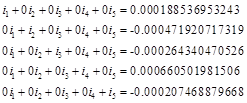(30)

Hence, from (30) we can isolate the circuit current as presented in (31) if format long was invoked during execution in MATLAB. For a corresponding solution with format short, we get the current in (32).(31)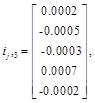(32)

Where j3 is the current obtained by method 3, Row-Reduced Echelon Method.

Substituting values of the obtained currents in (27) and (31) to check how they satisfy the values of v1 and v2 in (18) and (20) respectively gave the result in Table 1. Hence, the computation in Table 2-8.

Table 1. format long computed cases.

 Case I Case II Case III v1 = 100V v2 =1 V v1 = 100V v2 =100V v1 = 100V v2 =300V Matrix Inversion 99.99 0.99 99.99 100.00 99.99 300.00 Gaussian Elimination 99.99 0.99 99.9 100.00 99.99 300.00 Row-Reduced Echelon 100.0 1.00 99.99 100.00 99.99 299.86

Table 2. format short computed cases.

 Case IV Case V Case VI v1 = 100V v2 =1 V v1 = 100V v2 =100V v1 = 100V v2 =300V Matrix Inversion 100.00 1.01 100.00 100.00 102.00 305.00 Gaussian Elimination 100.00 1.01 100.00 100.00 102.00 305.00 Row-Reduced Echelon 106.00 10.00 104.50 95.00 102.00 305.00

Table 3. Single digit values for v2 with format short.

 Case VII Case VIII Case IX v1 = 100V v2 = 3 V v1 = 100V v2 = 5V v1 = 100V v2 = 7V Matrix Inversion 100.01 3.01 99.99 4.99 99.99 7.02 Gaussian Elimination 100.01 3.01 99.99 4.99 99.99 7.02 Row-Reduced Echelon 106 10 106 10 106 10

Table 4. Double digit values for v2 with format short.

 Case X Case XI Case XII v1 = 100V v2 =10 V v1 = 100V v2 = 30V v1 = 100V v2 = 70V Matrix Inversion 100.01 9.99 99.99 29.99 100.00 69.98 Gaussian Elimination 100.01 9.99 99.99 29.99 100.00 69.98 Row-Reduced Echelon 106 10 105.50 30 105 75

Table 5. Triple digit values for v2 with format short.

 Case XIII Case XIV Case XV v1 = 100V v2 =100 V v1 = 100V v2 = 200V v1 = 100V v2 = 270V Matrix Inversion 100.00 100 103.5 200 102.5 285 Gaussian Elimination 100.00 100 103.5 200 102.5 285 Row-Reduced Echelon 104.50 95 103.5 200 102.5 285

Table 6. Triple digit values for v2 with format short.

 Case XVI Case XVII Case XVIII v1 = 100V v2 =220 V v1 = 100V v2 = 260V v1 = 100V v2 = 285V Matrix Inversion 103 220 102.5 265 102 285 Gaussian Elimination 103 220 102.5 265 102 285 Row-Reduced Echelon 103 220 102.5 265 102 285

Table 7. Triple digit values for v2 with format short.

 Case XIX Case XX Case XXI v1 = 100V v2 =225 V v1 = 100V v2 = 265V v1 = 100V v2 = 275V Matrix Inversion 103 220 102.5 265 102.5 285 Gaussian Elimination 103 220 102.5 265 102.5 285 Row-Reduced Echelon 103 220 102.5 265 102.5 285

Table 8. Triple digit values for v2 with format long.

 Case XXII Case XXIII Case XXIV v1 = 100V v2 =220 V v1 = 100V v2 = 260V v1 = 100V v2 = 275V Matrix Inversion 99.99 220 100 260 100 275 Gaussian Elimination 99.99 220 100 260 100 275 Row-Reduced Echelon 99.99 220 100 259.91 99.97 275.1

To determine the relationship between v2 and R3 we will use (20). Substituting the current values obtained by Gaussian Elimination Method for v2 = 300 in (20) we got (33) and (34) using format long and format short respectively.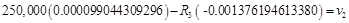(33)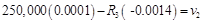(34)

For 150 ≤ R3 ≤ 250 kΩ, a plot of (33) and (34) gives the trend in Fig. 2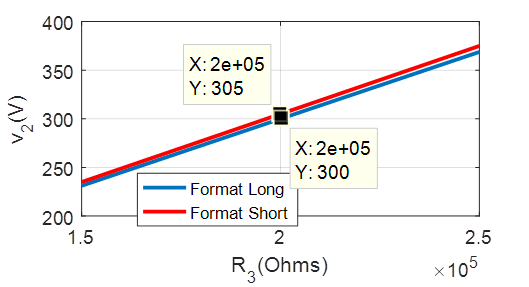Fig. 2. Allowable limit of v2 over a range of R3.

6. Discussion

Varying the circuit voltage v2 while ensuring that the circuit current does not exceed 0.001A, revealed possible solutions for positive values of v2 from 1-300V. We randomly selected 24 cases-leading to actually solving 24 set of linear algebraic system equations.

Condition for a satisfactory result for v1 and v2 for every solution is based on the fact that voltage difference or error meet the conditions in (35)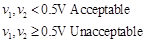(35)

In Table 1, the computation was such that the computed currents will be in 15 decimal places. Computed values of current substituted back into (18) and (20) gave voltages v1 and v2 with errors of 0.01V with Matrix Inversion and Gaussian Elimination Methods. While the largest error of 0.04V was observed in Case III with the Row-Reduced Echelon Method. Thus, any of the algorithm could be used in this situation for the selected voltages as a solution method because the errors are negligible.

Repeating the same computation such that all computed currents are truncated and rounded up to 5 decimal places, gave the result in Table 2. Gaussian Elimination and Matrix Inversion methods gave acceptable results in Case IV and Case V. In Case VI, errors of 2V with v1 and 5V with v2 were evident with all the three algorithms, this is unacceptable. In all 3 cases, Row-Reduced Echelon Method gave unacceptable errors of 6V, 4.5V and 2V for v1 and 10V, 5V and 5V for v2, respectively. Row-Reduced Echelon method in Case IV produced the largest error.

Based on the observed magnitude of error with Row-Reduced Echelon method in Case IV, we selected randomly single digit value for v2 to form the results in Table 3. Computing with format short, it was observed that the unacceptable errors of 6V with v1 and 10V with v2 was sustained all through when Row-Reduced Echelon Method was use. On the other hand, Gaussian Elimination and Matrix Inverse methods gave the same results with an average error of 0.01V, which is acceptable.

Increasing the values of v2 to a randomly selected double digit gave the result in Table 4. Here also, Gaussian Elimination and Matrix Inversion gave the same result, which are acceptable. Error plagued the Row-Reduced Echelon method again due to truncation and round-off. This is evident with all the Cases. The largest magnitude of error is evident in Case X, where v1 has an error of 6V while v2 has an error of 9V (90%).

When v1 and v2 have the same value, as seen in Case XIII of Table 5, both Matric Inversion and Gaussian Elimination Methods gave the same results and are acceptable. Row-Reduced Echelon Method produced errors of 4.V with v1 and 5V with v2, this is unacceptable. In cases XIV and XV, all three algorithms gave errors of 3.5V and 2.5V for v1 respectively. Disturbingly, about 15V was recorded as error in Case XV for v2. This is attributed to the value of currents i3 and i5 in Case XIV, the same values of current were also given by the computer due to truncation and round-off when the v2 increased from 200V to 270V.

In Table 6 and 7, all algorithms gave the same results. Voltage v1 values in Case XVI and XIX have 3V in excess. In Case XIX v2 was short of 5V. In Case XVII and Case XX excess voltages of 2.5V were observed with v1. Notice that v2 in Case XVII has an error of 5V while in Case XX no error is recorded with v2. Notice that in Case XXI, all three algorithms gave the same result with errors of 2.5 V for v1 and 10V for v2.

The voltages (v1 and v2) in Table 6 and Table 7 have the same values in all Cases. This should not be because v2 is changing. This is due to the values of i1,i3,i4 and i5 computed from Case XVI to Case XXI remains the same due to truncation and round-off.

To re-affirm the observation in Table 1, the results in Table 8 were presented. With format long (15-digit decimal place), all algorithms gave the same result with acceptable satisfaction of the system of equations.

It was also observed that when computed current values were substituted into (19), (21) and (22), very small negative values were obtained. It is implied that these values are just zero because in Fig. 1, only v1 and v2 exists.

The limiting v2 voltage for the circuit is 300V. Substituting current values obtained using format long and format short for this value of v2 gave (33) and (34) respectively. The effect of round-off and truncation was then depicted graphically in Fig. 2. It is clearly seen that the allowable range of v2 is increasing with increase in resistance R3. The challenge here is that with (34), this increase is expected to be sustained with at least 5V in excess or in error from the point R3 =200KΩ, v2 =300V.

Assessing the performance of the three algorithms, we can say that Row-Reduced Echelon Method had the worst performance. The need to investigate solutions to a system of algebraic equations by substituting back computed variables into the original equation cannot be over-emphasized. Results from this study have proved that if such substitution test is not done results obtained could be misleading.

7. Conclusion

In this study, numerical methods of Matrix Inversion, Gaussian Elimination and Row-Reduced Echelon methods were used to solve twenty-four (24) systems of linear algebraic equations. Solutions obtained for these system of linear algebraic equations were obtained in two forms; 15 decimal places (format long) and 5 decimal places (format short) in MATLAB. After obtaining the required solutions, we substituted them back into the equations to see if they were satisfied.

Six out of the twenty-four Cases (Case I-III, and Case XXII-XXIV) were computed using format long and all gave acceptable results with the three algorithms used. For the remaining eighteen (18) cases using format short, nine (9) gave acceptable results with Gaussian Elimination method and Matrix Inversion method (Case IV, V, VII, VIII, IX, X, XI, XII and XIII), while those of Row-reduced Echelon method were unacceptable. The remaining nine (9) cases gave unacceptable result with all three algorithms used (Case VI, XIV, XV, XVI, XVII, XVIII, XIX, XX, and XXI). The algorithm, Row-Reduced Echelon method gave unacceptable results with eighteen (18) Cases, hence not appropriate for the solution of this system of linear algebraic equation.

Gaussian Elimination method and Matrix Inversion method can be successfully used with either format long or format short values to obtain acceptable results with Case IV, V, VII, VIII, IX, X, XI, XII and XIII.

References

1. Howard Anton and Chris Rorres (2014). Elementary Linear Algebra: Application Version.ISBN 9781118434413.
2. David C. Lay, Steven R. Lay and Judi J. McDonald (2016). Linear Algebra and Its Applications, Fifth Edition. ISBN 978-0-321-98238-4.
3. David Poole (2011). Linear Algebra: A Modern Introduction. ISBN-13: 978-0-538-73545-2.
4. W.D Wallis (2012). A Beginners Guide to Finite Mathematics. ISBN 978-0-8176-8319-1.
5. Boege W et al (1986). Some Examples for Solving Systems of Algebraic Equations by Calculating Groebner Bases. University of Heidelberg, Institute for Applied Mathematics, Heidelberg, F.R.G. J. Symbolic Computation (1986) 1, 83-98.
6. Sohail A. Dianat and Eli S. Saber (2009). Advance Linear Algebra for Engineers with MATLAB. ISBN 13: 978-1-4200-9524-1.
7. Warren E. Stewart and Michael Caracotsios (2008). Computer-Aided Modeling of Reactive Systems. Copyright John Wiley & Sons, Inc.
8. Kenneth Hoffman and Ray Kunze (1971). Linear Algebra. Prentice-Hall, Inc.
9. William J. Palms III (2008). A Concise Introduction to MATLAB. ISBN 9780073385839.
10. Gilbert Strang (2005). Linear Algebra and Its Application. 4e, ISBN 13: 9780030105678.
11. Nicholas Loehr (2014). Advance Linear Algebra. ISBN-13: 978-1-4665-5902-8.
12. César Pérez López (2014). MATLAB Matrix Algebra. ISBN-13: 978-1-4842-0307-1.

 Contents 1. 2. 3. 4. 4.1. 4.2. 4.3. 5. 6. 7.
Article ToolsAbstractPDF(273K)